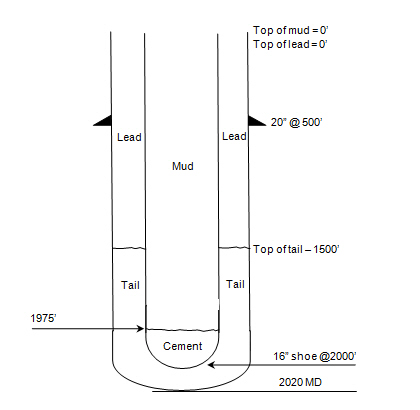# How much pressure will we see at cement head in case of float shoe fail?

I still have the simple but interesting question about hydrostatic pressure which you can apply this calculation into drilling/cementing operation. The question I got about how much pressure we will see at cement head in case of float shoe fail.Given situation as shown in figure above.  This situation is in vertical well.

1) Inside 16” shoe, from 0’ to 1975’ = 9.3 ppg mud

From 1975’ to 2000’ = 16.0 ppg mud

2) Outside 16” shoe, From 0’ to 1500’ = 11.6 ppg cement (lead)

From 1500’ to 2000’ = 16.0 ppg cement (tail)

Given the conditions above and assuming the cement is still liquid, how much pressure will we see at cement head in case of float shoe fail?

** Note : you need to understand how to calculate hydrostatic pressure in order to fully understand this question **

Using U-tube concept: Bottom hole pressure both sides are the same.

Let’s work out at annulus side which is heavier due to cement in the annulus

Pressure at bottom hole in annulus = hydrostatic pressure of lead cement + hydrostatic pressure of tail cement

Pressure at bottom hole  in annulus = 0.052×11.6×1500 + 0.052x16x(2000-1500) =1320.8 psi

Since, hydrostatic pressure in the annulus is more than hydrostatic pressure in 16″ casing; therefore, there will be pressure in the cement head in order to balance u-tube.

We can simply write equation as follows;

Bottom hole pressure = hydrostatic pressure inside 16” casing + surface pressure at cement head

1320.8 = 0.052x16x(2000-1975) + 0.052×9.3×1975 + surface pressure at cement head

Surface pressure at cement head = 1321 – 976 = 345 psi

Share the joyWorking in the oil field and loving to share knowledge.

### 3 Responses to How much pressure will we see at cement head in case of float shoe fail?

1.surender says:

I want to ask the very simple thing that if we use the Hydraulic Pressure, then easily we can have the result thorugh the machine.

why we need that process of calculating?

•DrillingFormulas.Com says:

Acutally, I would like to show how to apply concepts about hydrostaic pressure and U-tube concept. Understanding simply physical of these concepts, you can apply to serveral ways such as well control, completion fluid, cementing calculation, etc ; )

2.Gerard says:

Very good examples, it´s important to know how it works.
One question: The cementing pump should be working at least with 345 PSI, but as you go deeper the pump pressure should increasse? SInce the annulus column increases…

This site uses Akismet to reduce spam. Learn how your comment data is processed.